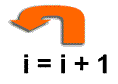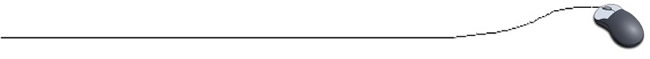Filling Variables

The equal sign (=) is used for assigning values to variables.
The format of an assignment is:
variable = expression;

The "variable" is a variable name that you defined in the program.
The "expression" is any variable, numerical/literal, or expression that produces a data type that is the same as the variable's data type.
 Data may be placed in a variable when it is declared: int height = 45; or data may be placed in a variable AFTER it has been declared (at a point further down in the program): int height; ... height = 45;Are computers and mathematicians always
speaking the same language?
A computer does not interpret an "equal sign" in the same manner that mathematicians do.  To a computer, the equal sign means that you want to take the number, variable, or expression on the right side of the equal sign and put it into the variable on the left.A computer understands:
but a computer does not understand
Remember that "98" cannot be the name of a variable location, since it starts with a number (9).The statement x = x + y; may look mathematically incorrect, but to a computer it means "take what was stored in x, add y to that value, and place the answer back in x".  Remember that what is on the right side will be STORED in the variable on the left.

Mathematician:i = i + 1   "NO way!!"
Computer:"Way cool!!"Answer:  While they agree on concepts, they do not necessarily agree on syntax (the manner in which the concepts are expressed).Compound Operators:

Java has its own shortcut syntax involving the placement of values into numerical variables.

x += y;  is the same as  x = x + y;
x -= y;  is the same as  x = x - y;
x *= y;  is the same as  x = x * y;
x /= y;  is the same as  x = x / y;
x %= y;
is the same as  x = x % y;

While a powerful tool used to update variables, compound operators can be troublesome.  In the order of operations, the compound operators have lower precedence than regular math operators.  Check out these two examples:

 int x = 42; int value = 0; value = value - x + 2; System.out.print(value); //gives - 40 int x = 42; int value = 0; value - = x + 2; System.out.print(value);  //gives - 44

Be careful when using compound operators.
Remember the operator precedence.